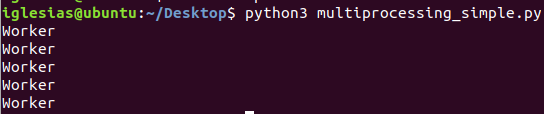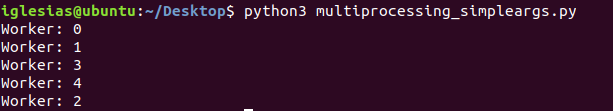# 第十章：使用进程、线程和协程提供并发性-multiprocessing:像线程一样管理进程-multiprocessing基础

10.4 multiprocessing:像线程一样管理进程

10.4.1 multiprocessing基础

import multiprocessing

def worker():
"""worker function"""
print('Worker')

if __name__ == '__main__':
jobs = []
for i in range(5):
p = multiprocessing.Process(target=worker)
jobs.append(p)
p.start()import multiprocessing

def worker(num):
print('Worker:',num)

if __name__ == '__main__':
jobs =[]
for i in range(5):
p = multiprocessing.Process(target=worker,args=(i,))
jobs.append(p)
p.start()©️2019 CSDN 皮肤主题: 技术黑板 设计师: CSDN官方博客